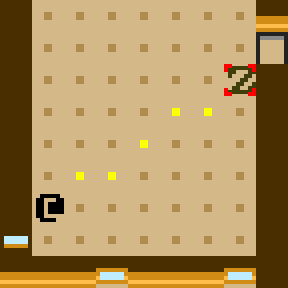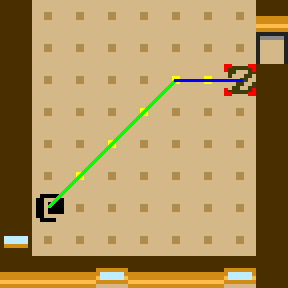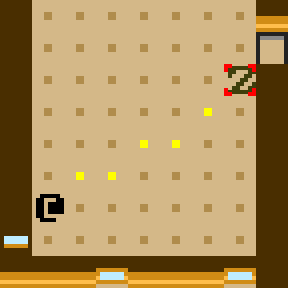# Even Separation Algorithm

This post describes an algorithm for evenly spreading out a sequence of items made up of two distinct types of item. I came up with it when attempting to draw straight lines on a grid, where lines are represented by discrete steps in one of two directions. In order for such a line to appear straight, the steps in one direction should be spread out as much as possible with respect to the steps in the other direction. The solution generalizes to spreading out any sequence made up of two distinct types of item that are repeated a number of times.Straight line from @ to Z, made up of steps to the east and northeast

## Straight Lines

In a 2D grid, a straight line is made up of steps in at most two directions. One of these is always a cardinal direction (north, east, south, west) and the other is an ordinal direction (northeast, southeast, southwest, northeast). It’s simple to determine the number of each steps required to get between two points. Suppose you take steps in the ordinal direction until you are in line with the destination in the cardinal direction, then move in the cardinal direction until you reach the destination.Splitting the line into its cardinal and ordinal components

To make the line appear straight, we have to spread out the cardinal and ordinal steps “as much as possible”. Being “as spread out as possible” turns out to be a non-trivial property to quantify. Assuming there are more ordinal steps than cardinal steps, there’s no reason to ever have two or more cardinal steps in a row. Thus the sequence of steps becomes groups of one or more ordinal steps, separated by individual cardinal steps. Also, we want the groups of ordinal steps to all be similar in size.First attempt at a straight line

The sequence of steps in the image above is: ⇗⇒⇗⇒⇗⇗. A close look reveals a slightly longer diagonal section at the Z end of the line. So what’s missing from the definition of “as spread out as possible”? How can we make this line more straight? This sequence can be thought of as groups of separated by . The sizes of these groups in the order they appear is: 1 1 2. What if we apply the same separation property to this sequence? There are more 1s than 2s, so no two 1s should be adjacent. Groups of 1s should be separated by individual 2s. Thus the sequence becomes: 1 2 1.Cardinal and ordinal steps are now as spread out as possible

The sequence of steps has become: ⇗⇒⇗⇗⇒⇗. Representing it as group sizes, it is: 1 2 1. This sequence of sizes can similarly be thought of as groups of 1s separated by 2s. The sequence of sizes of these groups is: 1 1. Now that we have a homogeneous list, there’s no further spreading necessary, and thus our sequence is as spread out as possible, and our line is as straight as possible.

## As spread out as possible

Let’s quantify the property that makes a sequence “as spread out as possible”. As you probably inferred from the above example, this property is recursive.

The first base case: A sequence is as spread out as possible if all its elements are homogeneous. E.g. AAAAAAAA

The second base case: A sequence is as spread out as possible if it is made up of an equal number of two distinct types of element, and the sequence alternates between elements one at a time. E.g. ABABABAB

The recursive case: A sequence is as spread out as possible if elements of its most-frequent type are arranged into groups separated by individual elements of its less-frequent type, such that:

• there are at most two different sizes of group
• if there are two different group sizes, the larger size is 1 greater than the smaller size
• the sequence of group sizes in the order they appear is as spread out as possible

## Separation Algorithm

This algorithm takes as input a pair of elements `a` and `b`, and non-negative integers `na` and `nb`, and returns a sequence containing `na` copies of `a`, and `nb` copies of `b`, that is as spread out as possible. The core idea is to figure out the group sizes and how many groups of each size will be present in the output, then recur with the group sizes and number of each group size as arguments. The result will be a sequence of group sizes that is as spread out as possible, that can then be used to construct a spread out list of elements.

``````function spread(a, b, na, nb) {

// allows us to assume na >= nb
if (na < nb) {
}

// first base case - no need for na == 0 case, as na >= nb
if (nb == 0) {
return [a, a, a, a, ...];
}

// second base case
if (na == nb) {
return [a, b, a, b, ...];
}

/* Because of the second base case, at this point we know
* that na > nb. Thus, the result will be groups of "a",
* separated by individual "b".
* E.g. aaa b aaaa b aaa b aaaa b aaa
*/

// sequence starts and ends with a group of "a"
let numGroups = nb + 1;

// there may be up to two group sizes
let smallGroupSize = floor(na / numGroups);
let largeGroupSize = smallGroupSize + 1;

/* To determine the number of small and large groups:
*
*      numGroups == numSmallGroups + numLargeGroups
*
*      na == numSmallGroups * smallGroupSize +
*            numLargeGroups * largeGroupSize
*
*         == numSmallGroups * smallGroupSize +
*            numLargeGroups * (smallGroupSize + 1)
*
*         == numSmallGroups * smallGroupSize +
*            numLargeGroups * smallGroupSize +
*            numLargeGroups
*
*         == smallGroupSize * (numSmallGroups +
*                              numLargeGroups) +
*            numLargeGroups
*
*         == smallGroupSize * numGroups +
*            numLargeGroups
*
*      numLargeGroups == na - numGroups * smallGroupSize
*/
let numLargeGroups = na - numGroups * smallGroupSize;
let numSmallGroups = numGroups - numLargeGroups;

/* In the "aaa b aaaa b aaa b aaaa b aaa" example:
* na == 17
* nb == 4
* numGroups == 5
* smallGroupSize == 3
* largeGroupSize == 4
* numSmallGroups == 3
* numLargeGroups == 2
*/

// recur on group sizes
numSmallGroups, numLargeGroups);

// total number of elements in result sequence
let nTotal = na + nb;

// create array to hold result
let sequence = new Array(nTotal);

// will be used as index into sequence
let index = 0;

// construct sequence from group sizes
for (let size of groupSizes) {

// insert the current group
for (let i = 0; i < size; ++i) {
sequence[index] = a;
++index;
}

// instert the individual separator
if (index < nTotal) {
sequence[index] = b;
++index;
}
}

return sequence;
}

``````

## Complexity

Let’s work out the worst case execution time in terms of the value of `n == na + nb` (ie. the total length of the requested sequence).

If we ignore the recursion for a second (pretend any recursive calls are instant), this algorithm includes a single loop, which iterates exactly `n` times as it builds up the result sequence. Thus the complexity of the algorithm ignoring recursive calls is linear.

When we recur, the length of the requested sequence is `numSmallGroups + numLargeGroups == numGroups == nb + 1`. Recall that in the non-trivial case, `nb` is strictly less than `na`. Thus, `nb + 1` is at most `n / 2`.

So the cost of the first recursive call (ignoring any further recursions) is `n / 2`. If this call makes further recursions, each will again cost at most half of the callers value of `n`. Thus, the total complexity can be expressed as ```O(n + n/2 + n/4 + n/8 + ...) == O(n * (1 + 1/2 + 1/4 + 1/8 + ...)) == O(n * 2) == O(n)```.